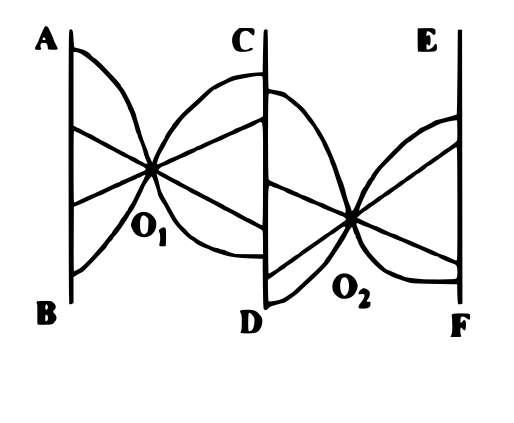Question 2

# Refer to the figure given below. AB, CD and EF are three parallel paths. A person starts from AB to reach EF by moving in four steps - moving from AB to $$O_1$$ in step 1, from $$O_1$$ to CD in step 2, from CD to $$O_2$$ in step 3 and from $$O_2$$ to EF in step 4. If he takes a curved path in one step, he cannot take a curved path in the next step. In how many ways the person can reach EF from AB ?Solution

The total number of possible ways from AB to EF = $$4\times 4\times 4\times 4=256$$

If a curved path is taken in a step, then the next step cannot be a curved path.

Let C denotes a curved path and S denotes a straight path.

Thus, the invalid paths are as follows: CCCC, CCCS, CCSC, CSCC, SCCC, CCSS, SCCS, SSCC. Thus, a total of 8 paths.

Total number of arrangements for these 8 paths = $$8\times 2\times 2\times 2\times 2=128$$

Thus, the total number of valid paths = $$256-128=128$$

Hence, the answer is option A.